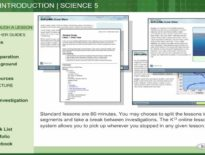# Online Learning Math: Probability Example

Interested in learning more about what online learning looks like? Use the animation below to experience a sample of how an online teacher uses technology to interact with her students and teach them about different ways to show fractions using a probability example.

*Note: This is just an example as students do have the opportunity to interact with the teacher which is not demonstrated in this activity.

This course can be found in our Fundamentals of Geometry and Algebra Course

Course Details:

Students enhance computational and problem-solving skills while learning topics in algebra, geometry, probability, and statistics. They solve expressions and equations in the context of perimeter, area, and volume problems while further developing computational skills with fractions and decimals. The study of plane and solid figures includes construction and transformations of figures. Also in the context of problem solving, students add, subtract, multiply, and divide positive and negative integers and solve problems involving ratios, proportions, and percents, including simple and compound interest, rates, discount, tax, and tip problems. They learn multiple representations for communicating information, such as graphs on the coordinate plane, statistical data and displays, as well as the results of probability and sampling experiments. They investigate patterns involving addition, multiplication, and exponents, and apply number theory and computation to mathematical puzzles.

## Online Learning Math: Probability Example